Michaela Fooksa, Jennifer Beddoe
• Author
Michaela Fooksa

Michaela received her Bachelor's degree in Mathematics from Colgate University and her Master's in Chemical and Physical Biology from Vanderbilt University. For the past two years, she has been teaching middle and high school math, physics, life science, and computer science.

• Instructor
Jennifer Beddoe

Jennifer has an MS in Chemistry and a BS in Biological Sciences.

Read the definition of a radian. Understand what a radian measure of an angle is, and learn how to find the radian measure using the radian formula. Discover how to convert a degree measure into a radian measure. Updated: 11/02/2021

Show

A radian is a unit of measurement of an angle that can be defined as the measure of an angle that subtends an arc with a length of exactly one radius. This definition will be explained in more detail below.

Review of Circle Terminology
The center of a circle is, as the name suggests, its exact center. A line drawn from the center to any point on the circle has the same length; that length is called the circle's radius. An arc is a part of the circle between any two points on the outside of the circle. In the image below, point A is the center of the circle. The line segments {eq}\overline{AB} {/eq} and {eq}\overline{AC} {/eq} are radii of the circle and have a length of 1. Thus, the radius of the circle is 1. The red curve connecting points B and C is an arc of the circle.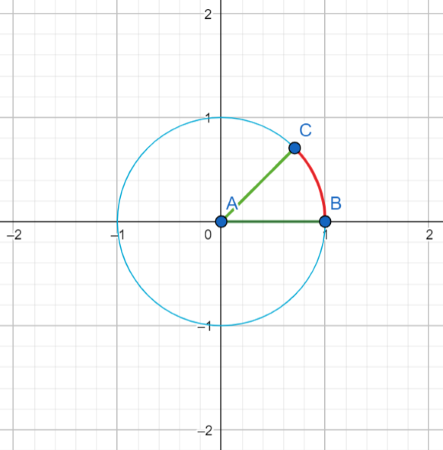What do these terms have to do with measuring an angle? Imagine in that same circle if the radius {eq}\overline{AB} {/eq} is taken and laid it along the circle.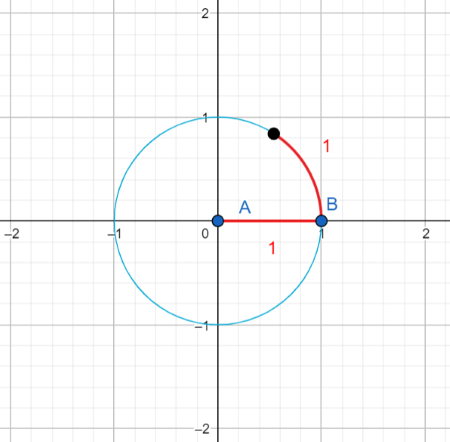The corresponding angle has a measure of exactly one radian, regardless of the radius of the circle.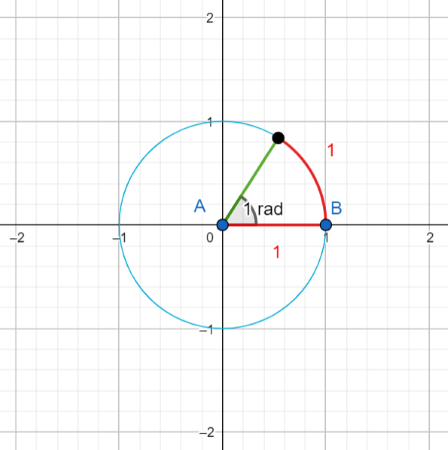Measuring angles in radians can be helpful in finding arc lengths of a circle. The formula for arc length is {eq}l=r\theta {/eq} where l is the arc length, r is the radius of the circle, and {eq}\theta {/eq} is the angle subtended by the arc.

For example, the arc length of the following circle can be found using this formula. The radius of the circle is 10 inches, and the angle subtended by the arc is 0.7 radians. Therefore, the length of the arc is {eq}l = r\theta = (10\, \mathrm{in})(0.7 \,\mathrm{ rad}) = 7 \,\mathrm{ in} {/eq}.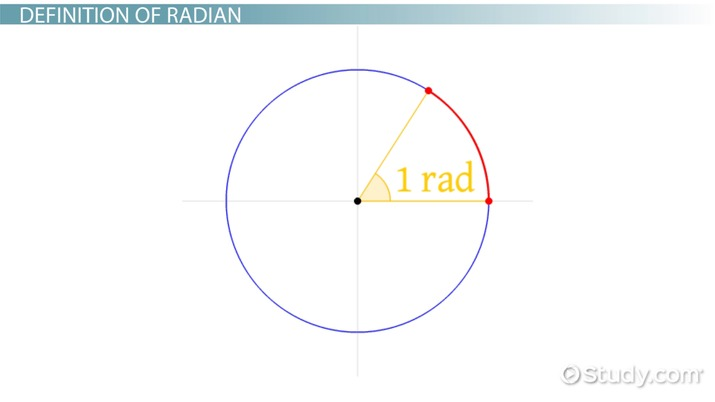An error occurred trying to load this video.

Try refreshing the page, or contact customer support.

Coming up next: Radians and Degrees: Definition & Examples

### You're on a roll. Keep up the good work!

Replay
Your next lesson will play in 10 seconds
• 0:58 Convert Degrees to Radians
• 1:39 Example Problems
• 3:25 Lesson Summary
Save Save

Want to watch this again later?

Timeline
Autoplay
Autoplay
Speed Speed

## How to Find the Radian Measure from a Degree Measure

A circle has {eq}2\pi {/eq} radians or 360°. Similarly, the angle measure of a straight line is {eq}\pi {/eq} radians or 180°. Using this equivalency, conversion between degrees and radians can be done using the following formula: {eq}\frac{\mathrm{rad}}{\pi}=\frac{\mathrm{deg}}{180} {/eq} where rad is the angle in radians and deg is the angle in degrees. This formula can be manipulated to get a simple formula for converting an angle in degrees to one in radians: {eq}\mathrm{ rad}=(\pi)\frac{\mathrm{deg}}{180} {/eq} and for converting an angle in radians to one in degrees: {eq}\mathrm{ deg}=(180)\frac{\mathrm{rad}}{\pi} {/eq}.

The following examples will go through using the radian measure formula and converting between radians and degrees.

### Example 1: Application of the Radian Equation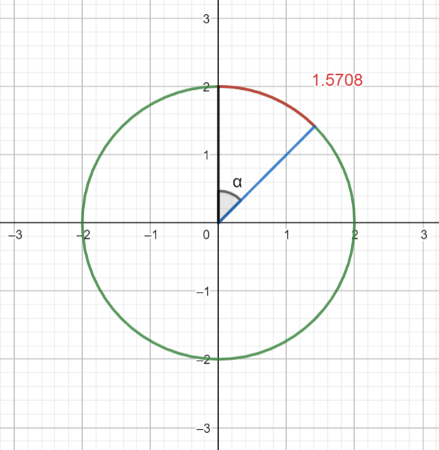In the above picture, angle {eq}\alpha {/eq} subtends an arc of length 1.5708 in a circle with radius 2. What is the measure of angle {eq}\alpha {/eq}?

The radian measure formula ({eq}l=r\theta {/eq} can be used to solve this problem. This formula can be rearranged to {eq}\theta=\frac{l}{r} {/eq} in order to solve for the angle {eq}\theta {/eq}. It is given that {eq}r = 2 {/eq} and {eq}l=1.5708 {/eq}. The unknown angle is {eq}\alpha {/eq}. Plugging in to the formula yields {eq}\alpha=\frac{1.5708}{2}=0.7854 {/eq}. Therefore, the measure of angle {eq}\alpha {/eq} is 0.7854 radians.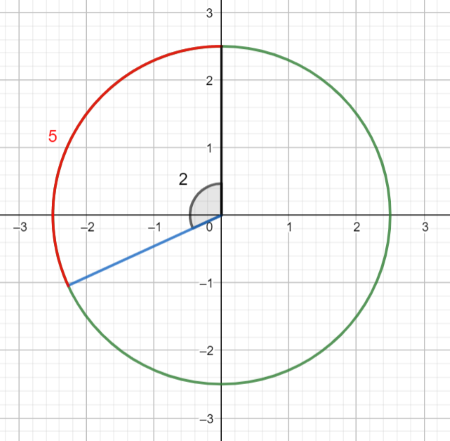To unlock this lesson you must be a Study.com Member.

#### How many radians are in a circle?

There are 2π radians in a circle. Since one radian subtends an arc with a length of r, the circle's radius, this means that the circumference of the circle is 2πr.

### Register to view this lesson

Are you a student or a teacher?